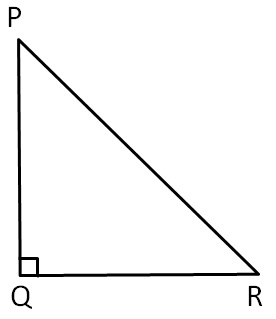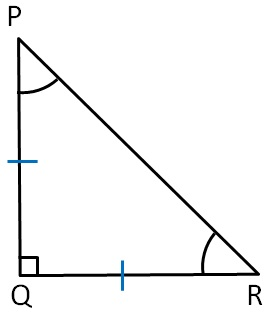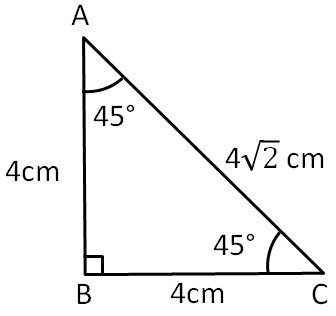Equilateral and Isoceles Triangle

Chapter 6 Class 7 Triangle and its Properties
Concept wise

In a right angled triangle , one angle is of 90°

and In isosceles triangle two sides are equal

Let us consider a right triangle ΔPQRNow,

For a right angled triangle PQR to be isosceles

We make two sides of a right triangle equal

Since PR is hypotenuse i.e. the longest side

Hence, it can’t be equal to any other side

Thus, other two sides are equal

∴ PQ = PRNow,

∠P = ∠R                 (Angles opposite to equal sides of a triangle are equal)

In ∆PQR, right angled at Q.

By angle Sum property,

∠P + ∠Q + ∠R = 180°

∠R + 90° + ∠R = 180°

2∠R = 180° − 90°

2∠R = 90°

∠R = (90°)/2

∠R =  45°

∴ ∠P = ∠R = 45°

Let’s find ∠ P and ∠ R

So, every right angled isosceles triangle has an angle of 90° and two angle of 45°.

For exampleLearn in your speed, with individual attention - Teachoo Maths 1-on-1 Class